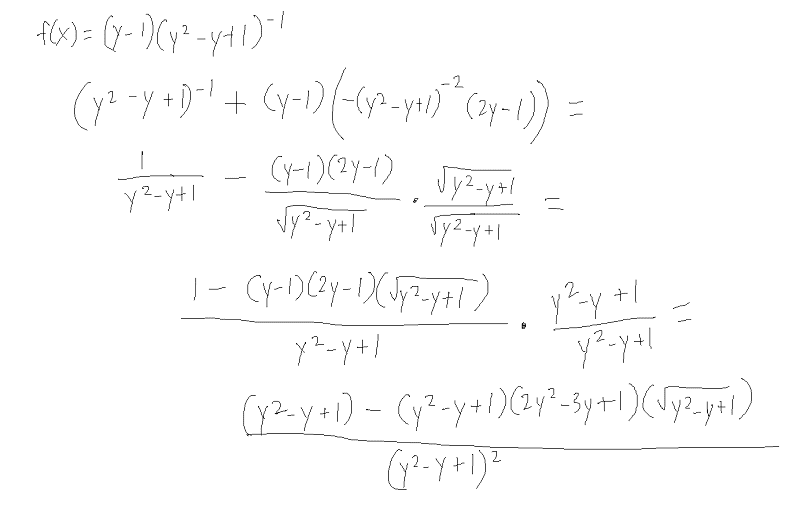# Trouble taking a derivative

PhizKid

## Homework Statement

Derivative of $$\frac{y - 1}{y^2 - y + 1}$$

## The Attempt at a SolutionThe solution is $$\frac{y^2 - 2y}{(y^2 - y + 1)^2}$$ but in my work, the answer will have something to the 4th power on the top which will be impossible to cancel out. What have I done wrong?

Edit: Never mind, I see my mistake

Last edited:

Scootertaj
Remember: $$\frac{d}{dx}\frac{f(x)}{g(x)} = \frac{g(x)f'(x) - f(x)g'(x)}{g(x)^2}$$

PhizKid
Yes but I don't like to work with the quotient rule. I should be getting the same answer using the product rule anyway, right?

Edit: Never mind, I see my mistake

Scootertaj
That's fine.

Then, remember: $$-(y^2-y+1)^{-2} = -\frac{1}{(y^2-y+1)^2}$$
(An expression to the -2 power doesn't equal 1/sqrt(expression))